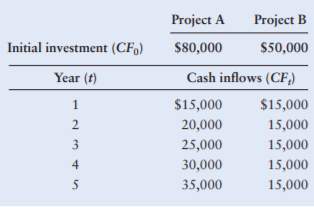### Create an Account

Already have account?

### Forgot Your Password ?

Home / Questions / All techniques with NPV profile Mutually exclusive projects Projects A and B of equal risk...

# All techniques with NPV profile Mutually exclusive projects Projects A and B of equal risk are alternatives for expanding Rosa Companys capacity The firms cost of capital is 13 The cash flows

All techniques with NPV profile—Mutually exclusive projects Projects A and B, of equal risk, are alternatives for expanding Rosa Company’s capacity. The firm’s cost of capital is 13%. The cash flows for each project are shown in the following table.
a. Calculate each project’s payback period.
b. Calculate the net present value (NPV) for each project.
c. Calculate the internal rate of return (IRR) for each project.
d. Draw the net present value profiles for both projects on the same set of axes, and discuss any conflict in ranking that may exist between NPV and IRR.
e. Summarize the preferences dictated by each measure, and indicate which project you would recommend. Explain whyJun 03 2021 View more View Less

#### Answer (UnSolved)Get Solution